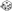General Use Thread - Page 38 - Myth-Weavers

 Notices The winners of the first Storycrafting contest have been announced! Congratulations to our grand prize winner, Underleaf, and the runners up!

# General Use Thread

Great Wyrm

Dice Roll: 1d20+8z 1d20+9z
d20 Results: 19 (Total = 27)
d20 Results: 6 (Total = 15)Spot and Listen (27,15)

Great Wyrm

Dice Roll: 1d20+4z
d20 Results: 20 (Total = 24)Perception (24)

Great Wyrm

Dice Roll: 1d20+8z 1d20+9z
d20 Results: 4 (Total = 12)
d20 Results: 6 (Total = 15)Spot and Listen (12,15)
Dice Roll: 1d20+2z
d20 Results: 18 (Total = 20)Heal (20)

Great Wyrm

Dice Roll: 1d20+9z 4d6+1z
d20 Results: 4 (Total = 13)
d6 Results: 4, 3, 3, 5 (Total = 16)Ranged Touch 1 (13,16)
Dice Roll: 1d20+9z 4d6+1z
d20 Results: 14 (Total = 23)
d6 Results: 1, 5, 1, 2 (Total = 10)Ranged Touch 2 (23,10)

Great Wyrm

Hellsing - Sense Motive

Dice Roll: 1d20+2z
d20 Results: 6 (Total = 8)

Great Wyrm

Dice Roll: 1d20+10z 2d6+6z
d20 Results: 17 (Total = 27)
d6 Results: 5, 6 (Total = 17)Greatsword AoO (27,17)
Dice Roll: 1d20+12z 2d6+6z
d20 Results: 2 (Total = 14)
d6 Results: 3, 5 (Total = 14)Greatsword with Flanking (14,14)

If applicable
Dice Roll: 1d20+12z 2d6+6z
d20 Results: 12 (Total = 24)
d6 Results: 1, 5 (Total = 12)Greatsword AoO on Minotaur standing up (24,12)

Great Wyrm

Dice Roll: 1d20+6z
d20 Results: 10 (Total = 16)Sense Motive (16)
These dice sets were omitted or moved: 1d8z

Great Wyrm

Dice Roll: 1d20+5z 1d20+3z
d20 Results: 5 (Total = 10)
d20 Results: 9 (Total = 12)Spot and Listen (10,12)

Wyrm

Dice Roll: 1d10z
d10 Results: 4Assensing (4)